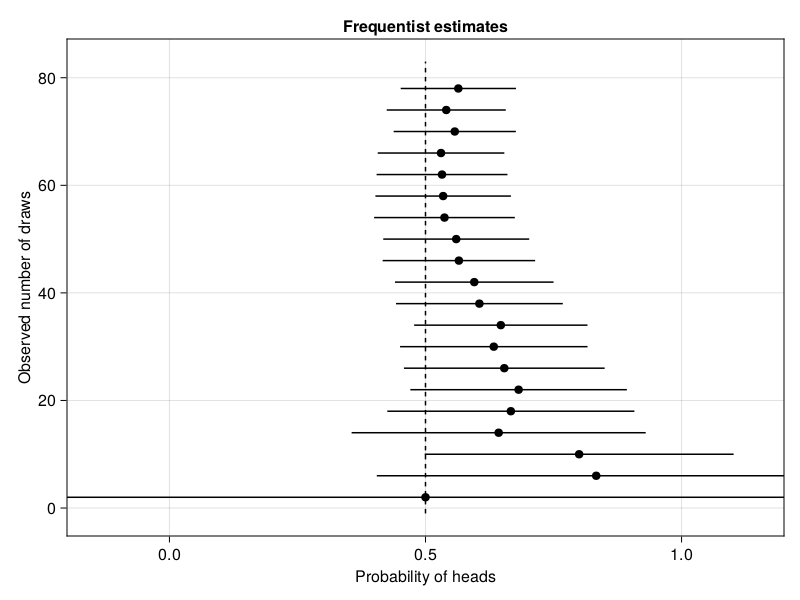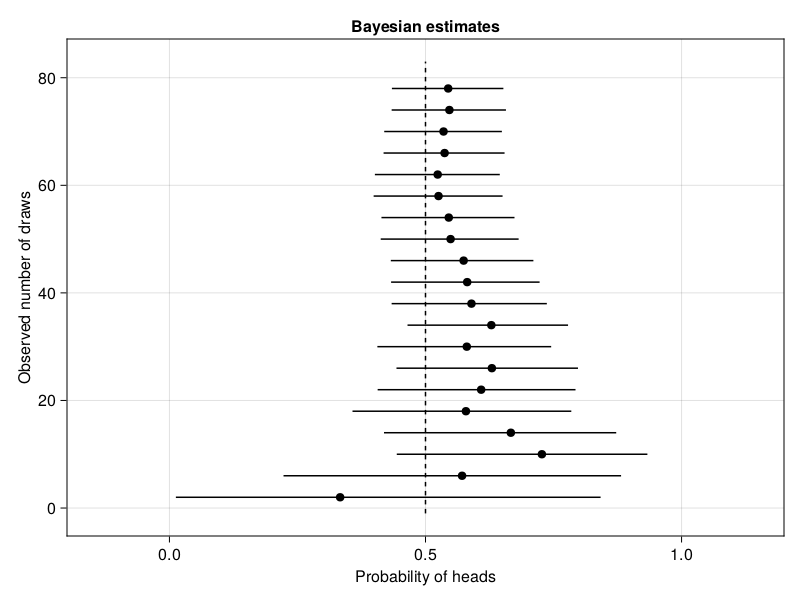HUIJZER.XYZ

# Frequentist and Bayesian coin flipping

2020-11-14

To me, it is still unclear what exactly is the difference between Frequentist and Bayesian statistics. Most explanations involve terms such as "likelihood", "uncertainty" and "prior probabilities". Here, I'm going to show the difference between both statistical paradigms by using a coin flipping example. In the examples, the effect of showing more data to both paradigms will be visualised.

## Generating data

Lets start by generating some data from a fair coin flip, that is, the probability of heads is 0.5.

begin
import CairoMakie

using AlgebraOfGraphics: Lines, Scatter, data, draw, visual, mapping
using Distributions
using HypothesisTests: OneSampleTTest, confint
using StableRNGs: StableRNG
end
n = 80;
p_true = 0.5;
is_heads = let
rng = StableRNG(19)
rand(rng, Bernoulli(p_true), n)
end;

To give some intuition about the sample, the first six elements of is_heads are:

is_heads[1:6]
6-element Vector{Bool}:
0
1
1
1
1
1

## Calculate probability estimates

The Frequentist estimate for a one sample t-test after seeing $n$ samples can be calculated with

function frequentist_estimate(n)
middle = t_result.xbar
lower, upper = confint(t_result)
return (; lower, middle, upper)
end;

For the Bayesian estimate, we can use the closed-form solution (https://turing.ml/dev/tutorials/00-introduction/). A closed-form solution is not available for many real-world problems, but quite useful for this example.

closed_form_prior = Beta(1, 1);
function update_belief(k)
updated_belief = Beta(closed_form_prior.α + heads, closed_form_prior.β + tails)
return updated_belief
end;
beliefs = [closed_form_prior; update_belief.(1:n)];
function bayesian_estimate(n)
distribution = beliefs[n]
q(x) = quantile(distribution, x)
lower = q(0.025)
middle = mean(distribution)
upper = q(0.975)
return (; lower, middle, upper)
end;

Now we can write a function to plot the estimates:

function plot_estimates(estimate_function; title="")
draws = 2:4:80
estimates = estimate_function.(draws)
middles = [t.middle for t in estimates]
lowers = [t.lower for t in estimates]
uppers = [t.upper for t in estimates]
df = (; draws, estimates, P=middles)
layers = data(df) * visual(Scatter)
df_middle = (; P=fill(0.5, length(draws) + 2), draws=[-1; draws; 83])
layers += data(df_middle) * visual(Lines) * visual(linestyle=:dash)
for (n, lower, upper) in zip(draws, lowers, uppers)
df_bounds = (; P=[lower, upper], draws=[n, n])
layers += data(df_bounds) * visual(Lines)
end

axis = (; yticks=0:20:80, limits=((-0.2, 1.2), nothing), title)
map = mapping(:P => "Probability of heads", :draws => "Observed number of draws")
draw(layers * map; axis)
end;

And plot the Frequentist and Bayesian estimates:

plot_estimates(frequentist_estimate; title="Frequentist estimates")plot_estimates(bayesian_estimate; title="Bayesian estimates")## Conclusion

Based on these plots, we can conclude two things. Firstly, the Bayesian approach provides better estimates for small sample sizes. The Bayesian approach successfully uses the fact that a probability should be between 0 and 1, which was given to the model via the Beta(1, 1) prior. For increasingly larger sample sizes, the difference between both statistical paradigms vanish in this situation. Secondly, collecting more and more samples until the result is significant is dangerous. This approach is called optional stopping. Around 10 samples, the frequentist' test would conclude that the data must come from a distribution with a mean higher than 0.5, whereas we know that this is false. Cumming (2011) calls this the "dance of the $p$-values".

EDIT: Christopher Rowley pointed out that it would be more fair to run a frequentist BinomialTest since that will output a confidence interval in [0, 1].

Built with Julia 1.9.3 and

AlgebraOfGraphics 0.6.14
CairoMakie 0.10.5
Distributions 0.25.93
HypothesisTests 0.10.13
StableRNGs 1.0.0

To run this blog post locally, open this notebook with Pluto.jl.